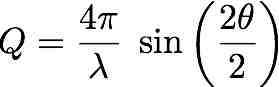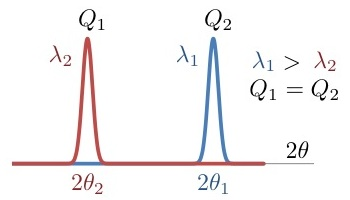# Simple scientific calculations

by Gabriel Cuello (2012) and A. Filhol (2018).

# Bragg's law

 Purpose: Given two of the three magnitudes (Q, lambda, 2theta) involved in the Bragg's law, it calculates the third one. Use: Check the radio button corresponding to the unknown magnitude and provide the other two. Example: lambda= 0.5 Å, 2theta= 30 degreesResult:  Q= 6.504832 Å-1Calculator
 Lambda = Å 2theta = deg Q = Å-1

top

# Scattering angles at different wavelengths

 Purpose: Given the observed scattering angle for a wavelength, it calculates the observed position at a different wavelength. It also calculates the corresponding modulus of the scattering vector. Use: Provide both the two wavelengths and the scattering angle for the wavelength 1. Example: At 2.52 Å a peak is observed at 20 degrees. At 1.28 Å the same peak will be observed at a smaller scattering angle.Result: New scattering angle: 10.12 degrees (for Q= 0.865924 Å-1)Calculator
 Lambda1 = Å Lambda2 = Å 2Theta1 = deg 2Theta2 = deg Q = Å-1

top

# Neutron Scattering Conversion Factors

 Purpose: Converts energies to different usual energy units in neutron scattering. Use: Check the radio button corresponding to the known unit and give its value. Example: lambda = 0.5 ÅResult:  E= 327.2168 meV, T= 3797.189 K, v= 7912.068 m/s, etc.

Calculator
 Lambda = Å Wavevector = Å-1 Velocity = m/s Temperature = K Energy = meV Energy = kJ/mol Frequency = THz Angular frequency = rad/s Optical wavenumber = cm-1

top

# Mass/number density

 Purpose: Given two of the three magnitudes (mass density, particle density and molar mass) involved in the particle to mass density conversion, it calculates the third magnitude. Use: Check the radio button corresponding to the unknown magnitude and provide the other two. Examples: For H2O, rho= 1 g/cm3, molar mass= 18 g/molResult: particle density= 0.033456 molecules/Å3 For Vanadium, rho= 6 g/cm3, molar mass= 50.9415 g/molResult: particle density= 0.07093 atoms/Å3

Calculator
 rho = g/cm3 n = 1/Å3 M = g/mol

top

# Binary mixtures: mass/particle fractions

 Purpose: Given the particle (mass) fraction of a binary mixture, it calculates the mass (particle) fraction. Use: Check the radio button corresponding to the known fraction (particle or mass) for component A. As input you must give the molar masses of each component and the known fraction of component A. The corresponding unknown fraction for component A and fractions for component B are calculated. Example: H2O/D2O mixture in mass: 100 mg of H2O + 150 mg of D2O. Mass fraction of H2O = 100/(100+150) = 0.4 Molar masses: 18 g/mol for H2O and 20 g/mol for D2O. Results: Mass fraction of D2O = 0.6, (H2O)0.4255(D2O)0.5745

Calculator
 A B Molar mass = g/mol Particle fraction = ni/(nA+nB) Mass fraction = mi/(mA+mB)

top

# Absorption cross section: energy dependence

 Purpose: Calculates the absorption cross section for a monoatomic system as function of energy. Use: The thermal absorption cross section (at 2200 m/s) must be provided, as well as the energy (velocity or wavelength) for the calculation. A 1/v dependence is assumed. Check the radio button corresponding to the energy unit and give the corresponding value. Example: E= 600 meV, Abs cross section (at 2200 m/s) = 5.08 bResult: Abs cross section (E)= 1.04313 b

Calculator
 v (m/s) E (meV) lambda (A) Value
 Thermal absorption cross section = b Absorption cross section = b

top

# Temperature conversion

 Purpose: Converts temperatures between Celsius, Kelvin and Fahrenheit. Celsius = Kelvin - 273.15 Fahrenheit = 1.8 Celsius + 32 Use: Check the radio button corresponding to the known unit and give the temperature. Example: T= 25 CResult:  T= 298.15 K, T= 77 F

Calculator
 Celsius = C Kelvin = K Fahrenheit = F